# Class 9 NCERT Solutions- Chapter 7 Triangles – Exercise 7.2

### (i) OB = OC                     (ii) AO bisects ∠A

Solution: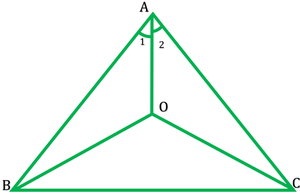Given: (i) An isosceles ∆ABC in which AB=AC

(ii) bisects of ∠B and ∠C each other at O.

Show: (i) OB=OC

(ii) AO bisects ∠A (∠1=∠2)

(i) In ∆ABC,

AB = AC

∠B =∠C                          [angles opposite to equal sides are equal]

1/2 ∠B = 1/2∠C

∠OBC=∠OCB

∴OB = OC                      [sides opposite equal ∠ are equal]

AB = AC                         [given side]
1/2 ∠B = 1/2∠C
∠ABO = ∠ACO               [Angle]
BO = OC                        [proved above side]
∴∆AOB ≅ AOC
Thus ∠1 = ∠2
Therefore, AO bisects ∠A

### Question 2. In ΔABC, AD is the perpendicular bisector of BC (see fig.). Show that ΔABC is an isosceles triangle in which AB = AC.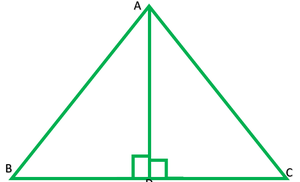Solution:

Given: AD is ⊥ bisector of BC

Show: AB=BC

In ∆ABD and ∆ACD

BD=DC                    [AD is ⊥ bisector side]

∴∆ABD≅∆ACD        [S.A.S]

AB=AC                     [C.P.C.T]

Question 3. ABC is an isosceles triangle in which altitudes BE and CF are drawn to equal sides AC and AB respectively (see Fig.). Show that these altitudes are equal.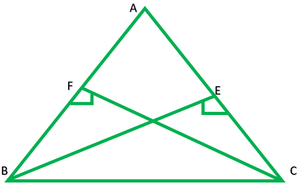Solution:

Given: AB=AC,BE and CF are altitudes

Show: BE=CF

In ∆AEB and ∆AFC,

∠E=∠F                     [Each 90° angle]

∠A=∠A                    [common angle]

AB=AC                    [given side]

∴∆AEB≅∆AFC        [A.A.S]

BE=CF                    [C.P.C.T]

### (ii) AB = AC, i.e., ABC is an isosceles triangle.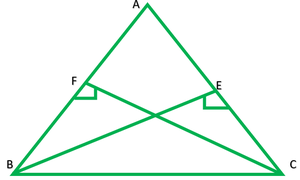Solution:

Given: Altitudes BE and CF to sides AC and AB are equal

Show: (i) ΔABE ≅ ΔACF

(ii) AB = AC

(i) In ∆ABF and ∆ACF,

∠E=∠F                    [Each 90° angle]

∠A=∠A                   [common angle]

AB=AC                   [given] S

∴∆AEB≅∆AFC       [A.A.S]

(ii) AB=AC             [C.P.C.T]

### Question 5. ABC and DBC are two isosceles triangles on the same base BC (see Fig.). Show that ∠ABD = ∠ACD.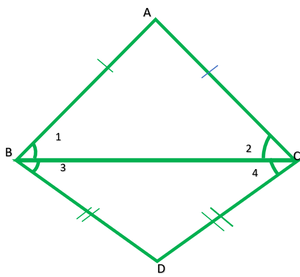Solution:

Given: AB=AC,BD=DC

Show: ∠ABD = ∠ACD

In ∆ABD,

∴∠1=∠2                  [angle opposite to equal sides are equal] 

In ∆BDC,

BD=DC

∴∠3=∠4                  [angle opposite to equal sides are equal] 

∠1+∠2= ∠2+∠4

∠ABD=∠ACD

### Question 6. ΔABC is an isosceles triangle in which AB = AC. Side BA is produced to D such that AD = AB (see Fig.). Show that ∠BCD is a right angle.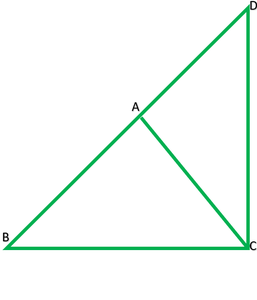Solution:

In ∆ABC,

AB=AC

∠ACB=∠ABC           

In ∆ACD,

∠BCD=∠ABC+∠BDC

∠BCD+∠BCD=∠ABC+∠BDC+∠BCD

2∠BCD=180°

∠BCD=(180°)/2=90°

### Question 7. ABC is a right-angled triangle in which ∠A = 90° and AB = AC. Find ∠B and ∠C.

Solution: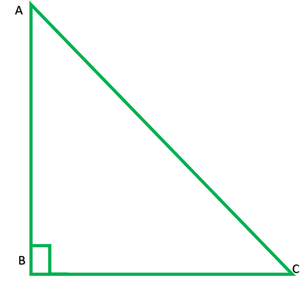Find: ∠B=? and ∠C?

In ∆ABC,

AB=AC

∴∠B=∠C                           [angle opposite to equal side are equal]

∠A+∠B+∠C=180°            [angle sum property of triangle]

90°+∠B+∠B=180°

2∠B=180°-90°

∠B=(90°)/2=45°

Therefore, ∠B=45° and ∠C =45°

### Question 8. Show that the angles of an equilateral triangle are 60° each.

Solution: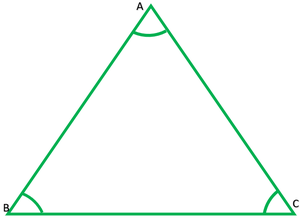Given: Let ∆ABC is an equilateral ∆

Show: ∠A=∠B=∠C=60°

In ∆ABC,

AB=AC

∠B=∠C                    

Also

AC=BC

∠B=∠A                    

From 1,2 and 2

∠A=∠B=∠C

In ∆ABC,

∠A+∠B+∠C=180°      [angle sum property of triangle]

∠A+∠A+∠A=180°

3∠A=180°

∠A=(180°)/3=60°

∠A=60°

∴∠B=60° and ∠C=60°

Whether you're preparing for your first job interview or aiming to upskill in this ever-evolving tech landscape, GeeksforGeeks Courses are your key to success. We provide top-quality content at affordable prices, all geared towards accelerating your growth in a time-bound manner. Join the millions we've already empowered, and we're here to do the same for you. Don't miss out - check it out now!

Previous
Next